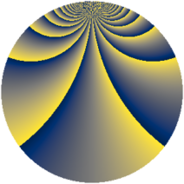# Properties

 Label 1045.2.ceLevel $1045$ Weight $2$ Character orbit 1045.ce Rep. character $\chi_{1045}(16,\cdot)$ Character field $\Q(\zeta_{45})$ Dimension $1920$ Sturm bound $240$

# Related objects

## Defining parameters

 Level: $$N$$ $$=$$ $$1045 = 5 \cdot 11 \cdot 19$$ Weight: $$k$$ $$=$$ $$2$$ Character orbit: $$[\chi]$$ $$=$$ 1045.ce (of order $$45$$ and degree $$24$$) Character conductor: $$\operatorname{cond}(\chi)$$ $$=$$ $$209$$ Character field: $$\Q(\zeta_{45})$$ Sturm bound: $$240$$

## Dimensions

The following table gives the dimensions of various subspaces of $$M_{2}(1045, [\chi])$$.

Total New Old
Modular forms 2976 1920 1056
Cusp forms 2784 1920 864
Eisenstein series 192 0 192

## Trace form

 $$1920 q + 12 q^{3} - 18 q^{6} + 36 q^{8} + 36 q^{9} + O(q^{10})$$ $$1920 q + 12 q^{3} - 18 q^{6} + 36 q^{8} + 36 q^{9} + 36 q^{14} - 36 q^{17} - 72 q^{18} - 24 q^{22} - 54 q^{24} + 36 q^{26} - 12 q^{27} + 18 q^{29} + 48 q^{30} - 12 q^{31} - 54 q^{33} - 216 q^{34} + 12 q^{35} - 246 q^{36} + 48 q^{37} + 24 q^{38} + 30 q^{41} - 294 q^{42} + 168 q^{44} - 96 q^{47} - 270 q^{48} + 216 q^{49} + 12 q^{50} + 48 q^{51} + 6 q^{52} + 72 q^{53} + 168 q^{54} + 144 q^{56} - 84 q^{57} - 96 q^{58} - 138 q^{59} + 72 q^{61} + 24 q^{62} + 240 q^{64} - 228 q^{65} - 66 q^{66} + 36 q^{67} - 84 q^{68} - 36 q^{69} + 216 q^{70} + 12 q^{71} - 72 q^{72} - 216 q^{73} + 12 q^{74} - 516 q^{76} - 144 q^{77} - 480 q^{78} + 456 q^{81} - 54 q^{82} - 72 q^{84} + 12 q^{86} - 48 q^{88} - 180 q^{89} + 48 q^{90} + 90 q^{91} + 48 q^{93} - 180 q^{94} + 192 q^{96} - 138 q^{97} - 264 q^{98} - 30 q^{99} + O(q^{100})$$

## Decomposition of $$S_{2}^{\mathrm{new}}(1045, [\chi])$$ into newform subspaces

The newforms in this space have not yet been added to the LMFDB.

## Decomposition of $$S_{2}^{\mathrm{old}}(1045, [\chi])$$ into lower level spaces

$$S_{2}^{\mathrm{old}}(1045, [\chi]) \cong$$ $$S_{2}^{\mathrm{new}}(209, [\chi])$$$$^{\oplus 2}$$